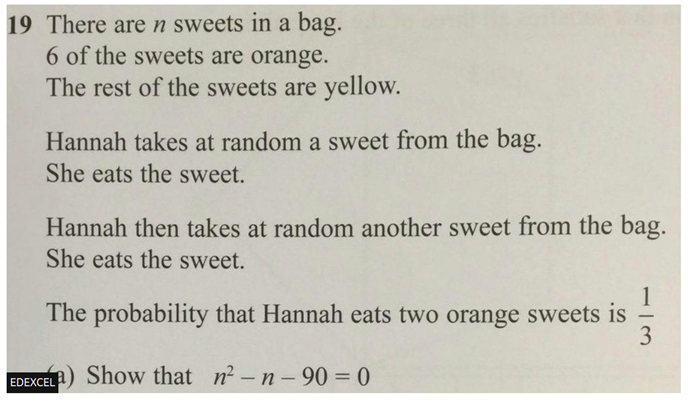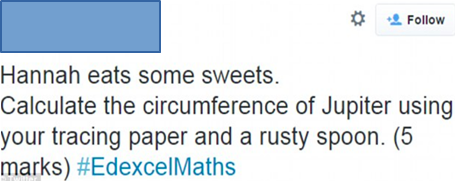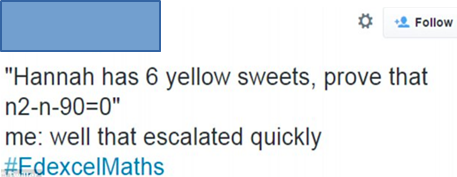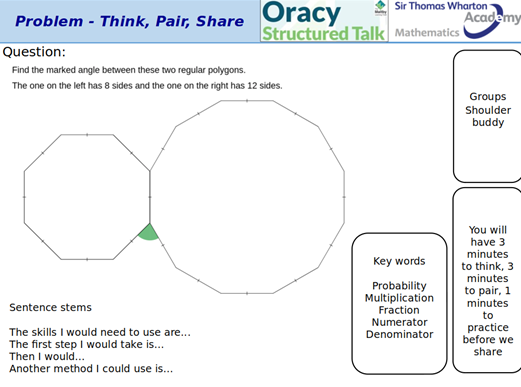# The importance of Mathematical IntuitionBlog by Joanne Hughes - mathematics teacher at Sir Thomas Wharton Academy with a Masters in Teaching and Learning.Never in GCSE mathematics has a question been discussed at such a length as ‘Hannah’s sweets’. This question brings reasoning and problem-solving skills together so that only those with strong mathematical intuition have a chance of answering it. Mathematical intuition is defined as:

‘...an extrapolation beyond the directly accessible information’ 

This links directly to ‘Hannah’s sweets’ question because it is not clear to students how they should proceed from probability to quadratics, shown by the following posts.This article discusses the importance of mathematical intuition in education and outlines the tasks that improve this. Intuition gained credibility in the 19th Century when academics began to identify the need to deepen students’ understanding of difficult mathematical questions. Bruner (1960) identified the importance of intuition in mathematics and science education, which was broadly missing in the schooling system. However, as mathematical intuition is a theoretical concept, there is a significant lack of research involving its application to teaching and learning (see Fischbein 1987 for further info).

Mathematical intuition enables students to attempt and understand reasoning and problem-solving questions. This skill supports students as they move to KS4 learning and has become increasingly important since the new GCSE specification was introduced in 2015. The introduction of questions, such as ‘Hannah’s sweets’, that do not lend themselves to following logical thought processes require mathematical intuition to succeed (Tall 1980, 1).

However, mathematical intuition is not only a skill that should be used during KS4 years to support their GCSEs. Including tasks and questions that aid students’ mathematical intuition at KS3 will ensure they have more time to improve their skills. Therefore, sooner is better.

How do we improve students’ mathematical intuition?

Integrating mathematical intuition into a streamlined task that could be taught to students is, unfortunately, impossible. However, academics have identified a range of specific tasks that give students the best possible chance to stimulate their mathematical intuition, and these are as follows:

1. Investigations

These can be whole lesson tasks or take no longer than 5 minutes. When a teacher introduces addition into their lesson with their year 7s, instead of modelling a basic question to the class and cold calling, they should ask the students what the sum of the numbers from 1 to 100 is [?]. This creates the shift where students are in control of their learning, leading to multiple answers.

Working with others is a great way to develop students’ mathematical intuition.  This is because it allows the students to draw on the understanding of one or two of their peers without directly relying on their teacher. The example is a great way to bring Oracy and reasoning tasks together.3. Reasoning

Reasoning is a skill that students need to be encouraged to do all the time. With an “explain the mistake task”, students deepen their understanding of topics and improve their intuition through application.

4. Problem-solving, having a guide

Often, with questions such as ‘Hannah’s sweets’, it is about getting the students to understand that there is no quick answer. It will take time to do the method, drawing from many topics along the way. The example is an excellent way of bringing oracy and problem-solving tasks together.The main factor

The ability for students to access their mathematical intuition by doing these tasks has one thing in common. It is the role of the teacher to create an environment in the classroom that encourages students to be engaged, want to know why, and understand that it is okay to make mistakes.

If there is one thing to take from this article, it is essential to constantly challenge the students you teach. Reasoning and problem-solving questions are a certainly a way of achieving this, however, teachers should not simply add these on a whim and expect instant results. Instead, they require commitment and understanding. Without these, students’ mathematical intuition has no chance of flourishing.

Bibliography

Bruner, Jerome, The process of Education, (USA: Harvard University, 1960)

Fischbein, Efraim, Intuition in Science and Mathematics: An Educational Approach, (Israel: Tel Aviv, 1987)

Hughes, Joanne, Research-based enquiry: Intuition in Mathematics, (UK: BCU, 2021)

Tall, David, ‘The Anatomy of a Discovery in Mathematics Research’ For the Learning of Mathematics, vol. 1, no. 2, (1980), pp. 25–34, <http://www.jstor.org/stable/40247713

    Jerome Bruner, The process of Education, (USA: Harvard University, 1960), pp. 55–68.

   Joanne Hughes, Research-based enquiry: Intuition in Mathematics, (UK: BCU, 2021) pp. 12–13.

 David Tall, ‘The Anatomy of a Discovery in Mathematics Research’ For the Learning of Mathematics, vol. 1, no. 2, (1980), pp. 25–34, <http://www.jstor.org/stable/40247713> (p. 1).

    Efraim Fischbein, Intuition in Science and Mathematics: An Educational Approach, (Israel: Tel Aviv, 1987), p. 13.